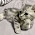## C Instruction With Example in C Language.

Hey Guys, So far we have covered some basic programs and things in c language. In this program, we are going to talk about instructions in c language. Let’s begin…

There are three types of instruction in c language:-
1. Type Declaration Instruction
2. Arithmetic Instruction
3. Control Instruction
Purpose of these instructions are below:-

1. Type Declaration Instruction:- Used to declare the type of variable
2. Arithmetic Instruction:- Used to perform the arithmetic operation
3. Control Instruction:- Used to control the sequence of the instructions.

Let us discuss this instruction in brief

1. Type Declaration Instruction

This Instruction is used to declare the type of variable which is used on the program. It is necessary to declare the variable first before using it on the program. The type declaration statement is written in the beginning of the main( ) function.

Ex :-

int abc;
float xyz;
char m, a, b;

(a)  You should also declare the variable value initially look at the example below

Ex :-

int x = 10,  y = 20,  z = 15;
float x = 17.5, y = 18.5, z = 20.256;

(b)  Always remember the order of the variable, sometimes the order of the variable is important, sometimes not. Look at the example below to see the difference

Ex:-

Int a = 10, b = 15;
It is same as
Int b = 15, a=10;
But
float a = 12.5, b = a + 4.15 ; is alright, but
float b = a + 4.15, a = 12.5 ; is not.

Because in the above declaration we are trying to use a variable before declaring it on the program.

2 Arithmetic Instructions

The general form of an arithmetic instruction follow rules given below.

i) It must contain one assignment operator  =.
ii) There should be one variable on the left-hand side of  =
iii) On the right side of =, there should be variables and constants.
iv) And those variables and constant will be connected by some arithmetic operators like +,-,*,/,%.

In C arithmetic instruction, it consists of a variable name on the left side to the = operator and variable name & constant on the right-hand side. The variables on the right-hand side of the = are connected with the arithmetic operator like = +,-,/,*; etc.

Ex:-

int  principle;
float  rate, time, simple_interest;
principle = 100000;
rate = 5.10;
time = 3.6;     /*3 year and 6 Months
simple_interest = principle * rate * time / 100;

Here,

• +,-,*,/ these are the arithmetic operators.
• = is the assignment operator.
• principle is an integer variable.
• rate, time, simple_interest are the real variable.
•  5.10, 3.6, are the real constant.
• 100000 is the integer constant.

What are operands?

Variables and constants together are called operands. These operands are connected by arithmetic operators.

3 Control Instruction

As the name suggests itself ‘Control Instructions’ enable us to specify the order in which the various instructions in a program are to be executed by the computer. In other words, the control instructions determine the ‘flow of control’ in a program.

There are four types of control instruction are:-

1. Sequence Control Instruction
2. Selection or Decision Control Instruction
3. Loop control instruction
4. Case-Control Instructions

In this tutorial, we learn about Instruction in C programming. If you find any mistakes on it. Or if you have any quarry related to it please comment below. Follow us for more programming tutorials.

You May Also Like

#### 1 comment:

1.👇
Kurulus Osman in English

📢Get high quality backlinks for your

Crypto quantum leap

📒 Read Home doctor book online
Then you will be a doctor for your family
Home Doctor Book

Tube Mastery and Monetization by matt

🦷Steel Bite Pro
Best product for
Teeth pain, cavities,teeth whitening and other oral health issues with money back guarantee
Steel Bite Pro